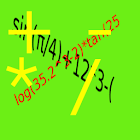EQ calc Pro

All Android applications categories

All Android games categories# EQ calc Pro

by: 0 0

## Screenshots

Description

Paid version of Equation entry/editable Calculator
Extended significant digit >16
Upper compatible to version1
No add,No limit.
You can save/load equation/contant on SD card by Menu!!

enter equation and Calculate.You can edit equation after many times.
If equation is too long.Touch equation and slide it.
Function calculation capable.
CLR:clear equation
BS:delete 1 char
Ans:set previous answer to equation
<>:enter edit mode and move cursor
ok:return to input mode
=:print answer

Tags: eq , calcpro , eq pro , eq calc , eqcalc